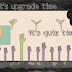(i) 36626337
(ii) 36626267
(iii) 36626762
(iv) 63378728

2.  0.44444 + 0.4444 + 0.444 + 0.44 + 0.4 = ?
(i) 18.7382
(ii) 21.7384
(iii) 22.7285
(iv) 2.17284

3. If  a/3 = b/4 = c/7, then the value of (a+b+c)/c is:-
(i) 1/7
(ii) 2
(iii) 7
(iv) none

4. If (2a+3b) (2c-3d) = (2a -3b) (2c-3d) then:-
(i) a/b =c/d
(ii) a/d = c/b
(iii) a/b =d/c
(iv) none

5. If 3x + 7 = x+ P = 7x + 5, what is the value of P.
(i)   1/2
(ii) 33/4
(iii) 17/2
(iv) can’t be determine

6.  x/y =6/5, the value of (x2+y2)/(x2-y2):-
(i) 54/11
(ii) 48/11
(iii) 61/11
(iv) 14/55

7. The value of (1 + 1/2)(1 + 1/3)(1 + 1/4)…………(1 + 1/120) is:-
(i) 30
(ii) 40.5
(iii) 60.5
(iv) 121

8. The value of  0.008 * 0.01 * 0.0072 /(0.12 * 0.0004) is :-
(i) 0.012
(ii) 0.12
(iii) 1.02
(iv) 1.2

9. 4.59 * 1.8/3.6+5.4 of 1/9-1/5 = ?
(i) 2.705
(ii) 2.695
(iii) 3.05
(iv) 3.009

10. (4335/4?244) /(15/8) = 289/528

(i) 1
(ii) 8
(iii) 0
(iv) 2
Answers will be updated at 9PM.
Attempt Free IIPB Pre Mock Test
Get Free Agriculture Officer Free Mock Test
Use Promocode CSA only for CSA, Kanpur Students and get 200 off on the complete Package.

Get The Offer
Math Quiz 2 for Bank/SSCReviewed by BasicKaMaster on 11:17:00 Rating: 5